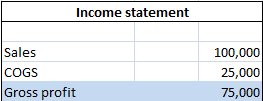# Gross Profit – Meaning and Formula

Gross profit also known as gross income is the excess of net sales over cost of goods sold. Put it simple, gross income is the difference between net revenue (net sale) and cost of goods sold. Gross income accounts for operating expenses, interests and taxes.

### Formula for the calculation of gross profit:

Gross income = Net sales – Cost of goods sold

Net revenue or sales is calculated by subtracting the amount of sales returns, allowances and discount from gross sales.

Net revenue = Gross sales – (Sales returns + Allowances + Discounts)

Cost of goods sold (COGS) refers to direct costs incurred in manufacturing products or providing services and is calculated as

COGS = Opening stock + Purchases – Closing stock

### Example:

From the figures given in income statement of XYZ ltd, gross income can calculated as:

###Gross sales = 100,000

Cost of goods sold = 25,000

Gross income = 1,00,000 – 25,000 = 75,000.

Gross income figure is used in calculation of one of the important profitability ratios known as gross profit margin.